Mysql underlying 啥 啥 use B tree without red black tree _MYSQL index underlayer data structure

What is an index?

1, the index is to help the database efficiently obtain the data structure of the data.

2, the index is stored in the file.

3, indexing is more than the effect of increasing the performance. (A table is allowed to allow 16 indexes)

(This picture below shows the computer composition principle. Each time the index node is queried, the disk IO read is performed, that is, seek and rotate)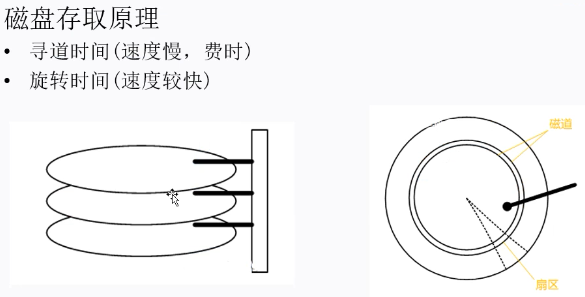Second, why is the mysql index structure B + tree?

Mysql builds an index can be used in the data structure of B + trees and hash, but Hash is very small, and the advantage is that it can quickly locate a row, and the disadvantage is that the range query problem cannot be resolved.

For a range of queries if you do not need to use a range query, you can use the Hash index method, such as check the phone number.

Let’s talk about the mainstream index method B + tree, let’s talk about why you don’t have to use other tree structure, then say why use B + tree.

1 Why don’t you use a binary tree?

If you encounter the extreme cases of the following single-sided growth, find node 4 and order lookups are not different. (This special case is equivalent to the linked list, the time complexity is O (n)))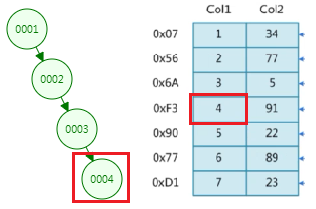2 Why don’t you need a red tree?

The red black tree is a balanced binary tree. When the amount of data is large, the depth of the tree is also very deep. If the depth of the tree has 20 layers, the data is found in the leaf node, it is necessary to carry out 20 IO operations, low performance .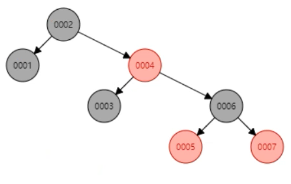3 Why don’t you use B?

B Tree Features:

The leaf node has the same depth

The pointer of the leaves node is empty

The data in the leaves node is incremented from left to right.

In fact, the B tree is in a horizontal, a node can store more data (large nodes contain a lot of small nodes), so relatively, the depth will become shallow.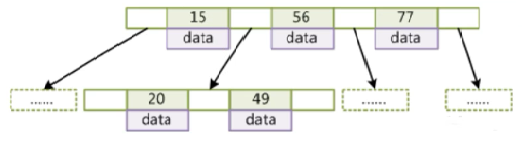Question: How to check the horizontal node, such as finding the node 77 in the figure?

From the disk, look out, load this large node into the memory, the node 77 is actually looking for in memory, and it is random access in memory, the speed is very fast, the disk is looking for If the rotation is compared, it can be basically ignored.

Question: Why can’t you make the B tree horizontally increase, this is not a depth of 1, and it’s faster? (The meaning of degree: Number of data stored in nodes)

Originally, I want to load a large node into memory through a large node, if the amount of data in a large node is too large, the memory and hard disk have no way to exchange so much data, and it is assumed to exchange 1 page (4K) The data (with the upper limit, also may be dozens of pages, and computer hardware), means that the CPU to do only one IO operation can only take 1 page data, then when a large number of data is too large, still To perform multiple IO operations. Therefore, the degree is capable, MySQL will automatically perform the degree optimization according to the computer hardware, and a large node is usually 1 space.

4 Why use B + tree? (B + tree is a variant of the B tree, index has been redundant, existed, but it doesn’t matter, the index only has a small space, such as 15 nodes in the picture below)

b + Tree Features:

The non-leaf node does not store DATA, only stores Key, which can increase the degree (compared to B + tree depth is more shallow)

Leaf node does not store pointers

Sequential access to the pointer, improve the performance of the interval access (actually two-way pointer)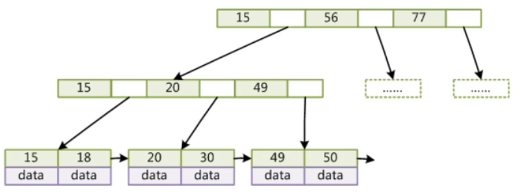Question: Why can the B + tree increase?

Because the non-leaf node only stores a value of the index, the DATA (B tree is stored), and the large node size is determined, so the large node can store more data, which means that it can become larger. This is guaranteed to reach the maximum, but also ensures that a large node can load into memory through the IO operation. (The non-leaf node is larger, the depth is shallow, only the non-leaf node affects the number of findings, the leaf node is the last lookup, which has no effect on the total look, so the DATA is moved to the leaves node)

Question: Why does I need a pointer between the leaf node? (The pointer connection between a large node’s tail node and the head node of the next large node)

Convenient range query. For example, find the key> 18 in the figure, if there is no pointer to be very troublesome, you must start from the beginning, if you have a pointer, you can directly traverse the Leaf node (Link list) of the key> 18.

B + tree index performance analysis:

General use of disk I / O number of evaluation index structures

Pre-reading: Disk is generally read sequentially, reads a certain length of data (integer times of pages) put into memory

Partial principle: When a data is used, the data near it usually uses immediately.

b + tree size size is set to equal to a page, each time the new big node directly applies a page space, which guarantees that a large node is physically stored in a page, and the large-scale load is only one IO operation.

B + tree degree d generally exceed 100, so the height H is very small (generally 3 ~ 5)

Third, how is the mysql underlying B + tree to store data?

Mysql has two common storage engines: InnoDB (default), Myisam (less, it is discarded in mysql8.0), and the storage engine range is a table level.

1, Myisam index implementation (non-aggregation)

Index files and data files are separated

The leaf node value of the index structure is stored in the file pointer.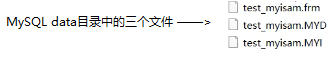.frm is a table structure file, .myd is a data file (MyISAM DATA) ,. MyiSam Index.

MYISAM Primary Key Arrow Find Process: Find the file pointer corresponding to the corresponding index first through the .myi file, and then locate the corresponding line according to the file pointer.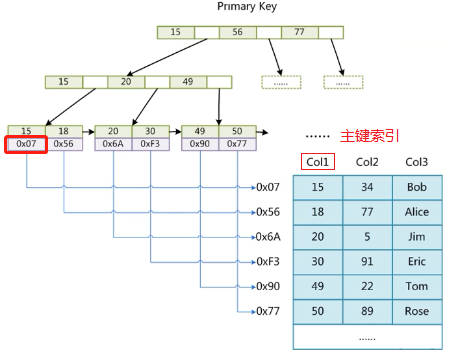MYISAM normal index lookup process: and the primary key index lookup process is consistent.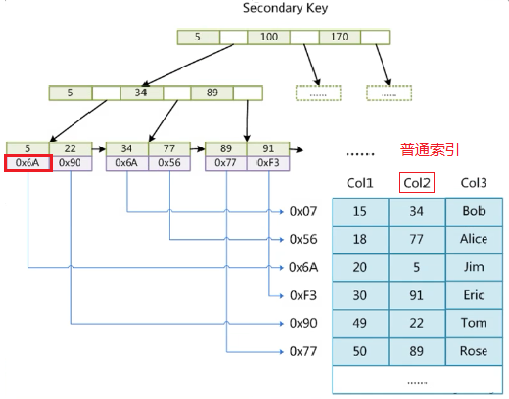2, InnoDB index implementation (gathering)

The data file itself is an index file.

The table data file itself is an index structure file that is organized by the B + tree.

The leaf node of the aggregated index contains a complete data record

The table must have a primary key, and it is recommended to use an integrated self-incrementary primary key.

The normal index structure leaf node stores the main key value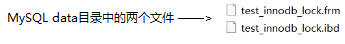.frm is a table structure file ,.ibd is a data and an index file (InnoDB Data)

Nodb primary key index lookup process: Find the corresponding index through .IBD file, the indexed value is the full data corresponding to the line.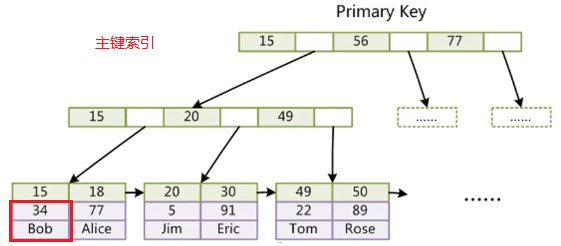inNodb Normal Index Finding Process: Find the corresponding index by .IBD file, the index value is the value of the primary key corresponding to the row, and then finds the corresponding row data in the primary key index tree.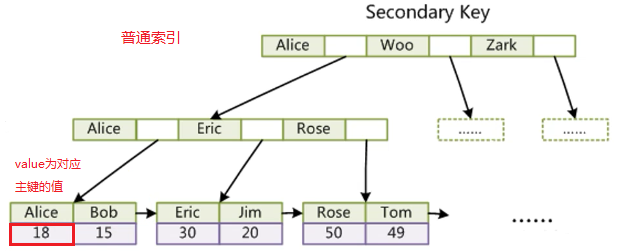Question: The difference between aggregation indexes and non-aggregated indexes?

Gathering Index: The index and data of the line data in the table are combined.

Non-aggregated index: The index and data of the line data in the table are stored separately.

Question: Why is the InnoDB table must have a primary key?

Because the entire data file itself is an index file organized in the B + tree, you must have a primary key (the primary key is not specified when the InnoDB is built. The default will select a column from the table field as the only master key, if this field does not exist, then there is no such field, then The background is generated by default a long integer key field, Myisam is not).

Question: Why is it recommended to use an integer self-incremental primary key?

Improve inquiry performance. If you use UUID as the primary key, the first, the UUID length is very long, wasting storage space, second, UUID is a string type, the bigger wants to find the ASCII code table, find the speed is not integrity INT lookup speed, third, UUID is a randomly generated string. When the data is inserted, it may cause the node position to move, which may also cause a lot of other node positions, simply, is disrupted. If you use an integer self-increment primary key, the newly inserted data will be continuously inserted into the physical space of the disk.

Question: Why is the INNODB normal index structure leaf node stores the main key value? (Consistency and save storage space)

If the Normal index value is also stored, then the value of the key corresponding to the index structure in the index structure is inserted into the data, and the maintenance cost is added to the index structure.

Single value index: there is only one index, such as (id), size = 1

Joint index: Multiple indexes are combined as a federated index, such as (id, name), size> 1 (single value index is a special case of combined index size = 1)

Question: What is the underlying data structure of the joint index?

(Leaf node Key is the combined index value, Value is a complete data of other fields other than the joint index)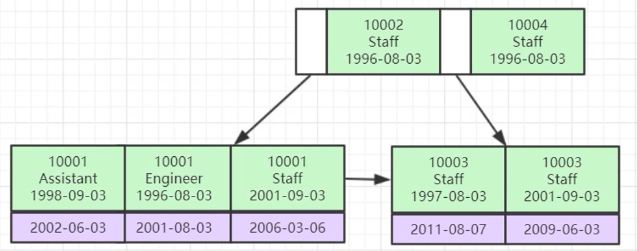First compare the ID, if the ID is equal, then compare the Name, if the Name is equal, then compare DATE. (Index the most left prefix principle, the back index optimization will explain)